×#### Thank you for registering.

One of our academic counsellors will contact you within 1 working day.

Click to Chat

1800-1023-196

+91-120-4616500

CART 0

• 0

MY CART (5)

Use Coupon: CART20 and get 20% off on all online Study Material

ITEM
DETAILS
MRP
DISCOUNT
FINAL PRICE
Total Price: Rs.

There are no items in this cart.
Continue Shopping```Methods of Integration

Table of Content

Integration by Substitution

Direct Substitution

Standard Substitutions

Indirect Substitutions

Related ResourcesWe have already discussed in the previous sections that various methods can be used for integrating functions. In case, the integrand is a derivative of a known function, the corresponding integral can be computed directly. But, in case the integrand is not a derivative of a known function then we might face certain issues as the integral cannot be computed directly.

Hence, in such problems where we need to find the integrals of some complex functions, we need certain special rules.

Basically, three methods of integration can be used while computing the indefinite integral of a function. These methods have been shown in the figure given below: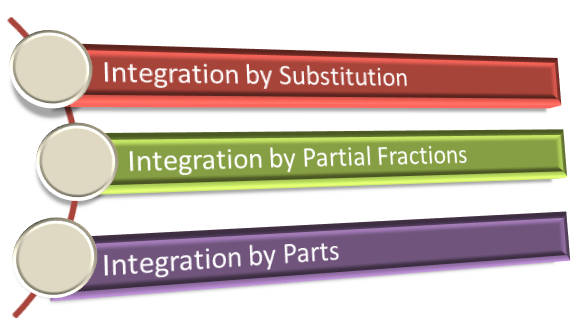Integration by parts and integration by partial fractions have been discussed in the later sections. In this section, our main emphasis would be on integration of functions using the substitution method.

Integration by Substitution

While solving integrals by the substitution method, some integrals can be computed using the direct substitutions while some need indirect substitutions. There are certain types of functions in which some standard substitutions are to be applied. Hence, in the substitution method, there are three major types of substitutions: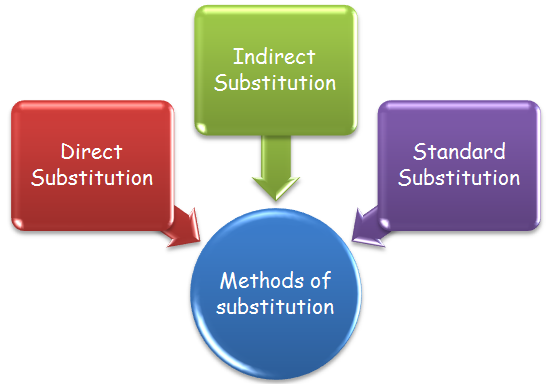We shall discuss all these methods in detail one by one. First of all we begin with the direct substitution method:

Direct Substitution

If the integral is of the form ∫ f(g(x)) g'(x) dx, then put g(x) = t, provided ∫ f(t) exists.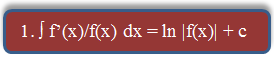The given integral is ∫ f'(x)/f(x) dx

Put f(x) = t ⇒ f'(x) dx = dt

⇒ ∫ dt/t  = ln |t| + c = ln |f (x)| + c.The given integral is ∫ f'(x)/√f(x) dx

Put f (x) = t

Then the given integral becomes

= ∫ dt/√t = 2 √t + c = 2 √f(x) + c.

We now discuss certain examples based on direct substitution:

Example 1:

Evaluate ∫ sin (In x)/x dx.

Solution:

The given integral is I = ∫ sin (In x)/x dx.

Let ln x = t. Then dt = 1/x dx

Hence I = ∫ sin t dt = – cos t + c = – cos (ln x) + c

_______________________________________________________________________________

Example 2:

Evaluate ∫ (2x2 + 1) sin2 (2x3 + 3x) dx.

Solution:

Let z = 2x3 + 3x

dz = (6x2 +3)dx = 3( 2x2 +1)dx

= 1/3 ∫ sin2z dz = 1/3 ∫ 1–cos2z/2 dz

= 1/6 ∫ (1–cos2z)dz = 1/6 [z – sin2z / z] + c

= 1/6 [2x2 + 3x –sin(4x3 + 6x)/2] = + cStandard Substitutions

In certain standard integrands, some standard substitutions prove helpful:

For terms of the form (x2 + a2) or √(x2 + a2), put x = a tan θ or a cot θ

For terms of the form (x2 - a2) or √(x2 – a2), put x = a sec θ or a cosec θ

For terms of the form (a2 - x2) or √(x2 + a2), put x = a sin θ or a cos θ

If both √(a+x), √(a–x) are present, then put x = a cos 2θ.

For terms of the type 2x/(a2 - x2), 2x/(x2 + a2), (x2 - a2)/(x2 + a2),the substitution x = a tan θ proves useful.

For the type √(x–a)(b–x), put x = a cos2θ + b sin2θ

For the type (√(x2 + a2)  ± x)n or (x ± √(x2 - a2)n, put the expression within the bracket = t.

For the type(n ∈ N, n > 1), put (x + b)/(x + a) = t

For 1/(x + a)n1 (x + b)n2, n1, n2 ∈ N (and > 1), again put (x + a) = t(x + b)

We now discuss some illustrations based on application of these substitutions:

Example 1:

Evaluate ∫ dx/(x + 1)6/5 (x – 3)4/5

Solution:

The given integral is I = ∫ dx/(x + 1)6/5 (x – 3)4/5

= ∫ dx / [(x + 1)2{(x – 3)/(x +1)}4/5]

Now put (x-3)/(x+1) = t

This gives dt = 4/(x+1)2 dx

Hence, I = ¼  ∫ dt / t4/5 = 5/4 t1/5 + c

= 5/4 {(x-3)/(x+1)}1/5 + c.

Indirect Substitutions

If the integrand f(x) can be written as the product of two functions of the form f(x)g(x), where g(x) is a function of the integral of f(x), then put integral of f(x) = t.

If ∫ f(x) dx = g(x), then ∫ f(ax + b) dx = 1/a g(ax + b)

∫ f’(x)/ f(x) dx = log {f(x)} + c

∫ f’(x)/√f(x) dx = 2√f(x) + c

∫ [f(x)]n f’(x) dx  = [f(x)]n+1/(n+1) + C, n ≠ 1

In order to evaluate integrals of the form ∫ sinmx dx,  ∫ cosmx dx where m ≤ 4, we express sinmx, cosmx in terms of sines and cosines of multiples of x by using trigonometric identities for sin2x, cos2x, sin 3x and cos 3x.

While evaluating integrals of the form sinmx cosnx dx, m, n ∈ N:

First check the exponents of sin x and cos x.

If the exponent of sin x is an odd positive integer put cos x = t

If the exponent of cos x is an odd positive integer put sin x = t

If the exponents of sin x and cos x both are odd positive integers put either sin x or cos x = t

If the exponents of sin x and cos x both are even positive integers then express sinmx cosnx in terms of sines and cosines of multiples of x by using trigonometric results of De’Moivre’s theorem.

Example 1:

Evaluate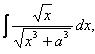Solution:

We first compute the integral of the numerator

The Integral of the numerator = x3/2 /(3/2)

Put x3/2 = t.

We get I = 2/3 ∫dt/√(t2 + a3)

= 2/3 In |x3/2 + √x3 + a3| + c.

Remark:

Sometimes it is useful to write the integral as a sum of two related integrals which can be evaluated by making suitable substitutions.

Examples of such integrals are:

A. Algebraic Twins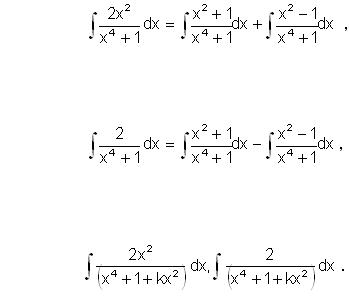B. Trigonometric twins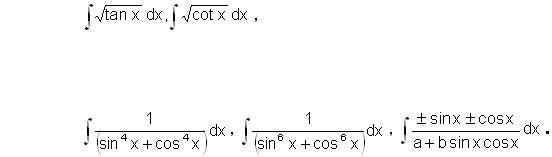Method of evaluating these integrals is illustrated by means of the following examples:

Example 1:

Evaluate ∫ 5/(1+x4) dx.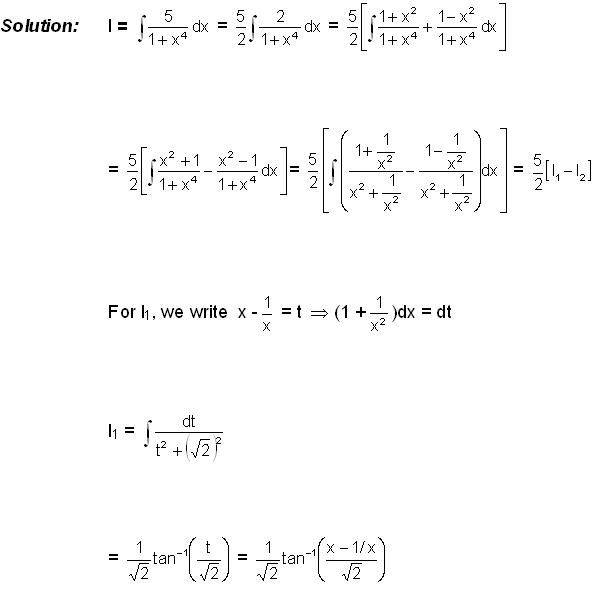For I2, we write (x + 1/x) = t ⇒ (1 – 1/x2)dx = dt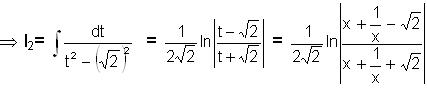Combining the two integrals, we get__________________________________________________________________________

Example 2:

Evaluate ∫ √tan x dx.

Solution:

Put tan x = t2 ⇒ sec2x dx = 2t dt

⇒ dx = 2t dt/(1 + t4)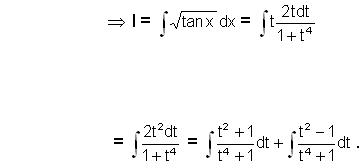This integral can be computed on similar lines as discussed in the previous example.Q1. In integrals of the form √(x–a)(b–x), the substitution that proves useful is

(a)  x = a cos2θ + b sin2θ

(b) put x = a cos θ and solve

(c  put x = sec θ + cosec θ

(d) none of the above

Q2. In case of integrals of the form (a2 - x2) or √(x2 + a2),

(a) use x = a sin θ only

(b) can use x = a cos θ only

(c) may use x = a sin θ or a cos θ

(d) x  = a sin θ + a cos θ

Q3. For terms of the type 2x/(a2 - x2), 2x/(x2 + a2), (x2 - a2)/(x2 + a2)

(a) use x = a sin θ only

(b) can use x = a cos θ only

(c) may use x = a sin θ or a cos θ

(d) x  = a tan θ

Q4. While evaluating integrals of the form sinmx cosnx dx, where the exponent of cos x is an odd positive integer one must

(a) sin x  = t

(b) sin x – cos x = t

(c) sin x + cos x = t

(d) cos x = t

Q5. For 1/(x + a)n1 (x + b)n2, n1, n2 ∈ N (and > 1),

(a) put (x + b) = t(x + a)

(b)  put (x + a) = t(x + b)

(c) put (x + a)2 = t(x + b)

(d) put (x + a) = t(x + b)2Q1.

Q2.

Q3.

Q4.

Q5.

(a)

(c)

(d)

(a)

(b)

Related Resources

You may wish to refer indefinite integral.

For getting an idea of the type of questions asked, refer the previous year papers.

To read more, Buy study materials of Indefinite integral comprising study notes, revision notes, video lectures, previous year solved questions etc. Also browse for more study materials on Mathematics here.
```### Course Features

• 731 Video Lectures
• Revision Notes
• Previous Year Papers
• Mind Map
• Study Planner
• NCERT Solutions
• Discussion Forum
• Test paper with Video Solution